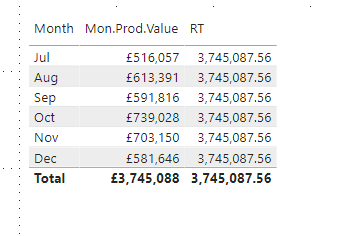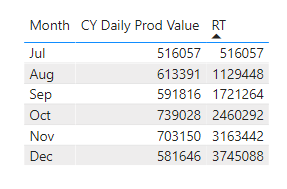cancel
Showing results for
Did you mean:Frequent Visitor

## Running Total Help

Hi All

Still trying to get to grips with some basic aspects of PowerBI.

I've a simple data model in play:  a date table and a daily production table with relationship between Dates[CurDate] and DailyProd[pdate].

I've created a measure that shows the monthly production values for the current fiscal year (starts in July).

This is the measure :

``````CY Daily Prod Value =
VAR MAXPDATE = maxx(allselected(DailyProd), DailyProd[pdate])
VAR CM_EOM = EOMONTH(MAXPDATE,0)
VAR PYear = year(MAXPDATE)
VAR FYear = calculate(min(Dates[FiscalYear]),Dates[CurDate]=MAXPDATE)
VAR PMonth = month(MAXPDATE)
VAR FirstDay = calculate(min(Dates[CurDate]),dates[FiscalYear]=FYear)
Return
CALCULATE([Daily Prod Value],
DATESBETWEEN(DailyProd[pdate],FirstDay,CM_EOM))``````

So this bit works.

I'm now trying to create a running total measure and have got as far as this:

``````RT = IF ([CY Daily Prod Value] <> BLANK(),
CALCULATE(
[CY Daily Prod Value],
FILTER(
ALLSELECTED(Dates),
Dates[CurDate] <= MAX( Dates[CurDate] )
)
)
)``````

This gives me the total of the Jul-Dec values but against each month!Any help (again) would be most appreciated.

Jake

1 ACCEPTED SOLUTIONFrequent Visitor

Hi All

I believe I have found a nice solution to my query.

I came across this great article https://www.sqlbi.com/articles/hiding-future-dates-for-calculations-in-dax/

So I followed the 2 methods described.

I created a calculated column in the Dates table:

``````DatesWithProdVals =
'Dates'[CurDate] <= MAX ( DailyProd[pdate] )``````

This column had a value of True up to and including the maximum production date.

I then created the following measure:

``````Sales YTD v1 =
CALCULATE (
[CY Daily Prod Value],
CALCULATETABLE (
DATESYTD ( Dates[CurDate],"30/06" ),
Dates[DatesWithProdVals] = TRUE
)
)``````

This measure gave me exactly what i wished.

I also followed the described procedure without creating a calculated column:

``````Sales YTD v2 =
VAR LastDayAvailable =
CALCULATE (
MAX ( DailyProd[pdate] ),
ALL ( DailyProd )
)
VAR FirstDayInSelection =
MIN ( 'Dates'[CurDate] )
VAR ShowData =
(FirstDayInSelection <= LastDayAvailable)
RETURN
IF (
ShowData,
CALCULATE (
[CY Daily Prod Value],
DATESYTD ( 'Dates'[CurDate],"30/06" )
)
)``````

This again produced the running ytd values for only July - December.  However this measure does not display a total value (see table below).  I don't know why.YTD Table

Thanks everyone for you help.

Until the next time 😉

Jake

7 REPLIES 7Frequent Visitor

Hi All

Sorry for the delay in getting back - I took a few days off for the holidays.

A bit of background.

I thought I'd start my PBI journey with by creating a report that displays the current monthly production values against budget and to also show the month by month production values for the current fiscal year again against budgets.  I thought that this would be simple (based on me being able to create this in SQL in a few minutes).

Since my last reply I started over and I have now got a version that is working but I'd like you all to have a look and critique.

The data model has only 2 tables: a date table, Dates, (marked as such) and a daily production table, DailyProd.

As this report will only show monthly/fiscal yearly data based on the last production date there are no filters/slicers on the page.

All the ** bleep** in the code is 3 letter abbreviation for Cumulative.

The first measure I created is:

``Daily Prod Value = sum(DailyProd[day_sales])``

then a cumulative daily production value measure:

``````**bleep** Daily Prod Value =
calculate(sum(DailyProd[day_sales]),
Dates[CurDate]<=max(Dates[CurDate]))``````

Next I created a measure to filter only month in which latest production value is:

``````CM Daily Prod Value =
var maxpdate = MAXX(allselected(DailyProd),DailyProd[pdate])
var CM_EOM =eomonth(maxpdate,0)
var FirstDay = date(year(maxpdate),MONTH(maxpdate),1)
RETURN
CALCULATE([Daily Prod Value],
filter(all(DailyProd[pdate]),
DailyProd[pdate] >= FirstDay && DailyProd[pdate]<= CM_EOM)
)``````

I then ended up creating 2 measures to create a running monthly total by day:

``````**bleep** CM Daily Prod Value =
CALCULATE([CM Daily Prod Value],
Dates[CurDate]<=max(Dates[CurDate])

)``````

The issue I had here was that the measure calculated a running total up to the maxium day in the dates table not just to the end of current production month.  I tried to add various filters etc to the measure but failed. So I then created one additional measure to restrict the output to only 1 month:

``````**bleep** CM Daily Prod Value CM =
var maxpdate = MAXX(allselected(DailyProd),DailyProd[pdate])
var CM_EOM =eomonth(maxpdate,0)
var FirstDay = date(year(maxpdate),MONTH(maxpdate),1)
RETURN
calculate([**bleep** CM Daily Prod Value],filter(Dates,Dates[CurDate]>=FirstDay && Dates[CurDate]<=CM_EOM))``````

Is it possible to do this filtering in 1st measure?

Fiscal Year

I created the following measure:

``````CY Daily Prod Value =
CALCULATE([Daily Prod Value],
FILTER(all(Dates[FiscalYear]),
Dates[FiscalYear]=calculate(max(Dates[FiscalYear]),Dates[CurDate]=maxx(allselected(DailyProd[pdate]),DailyProd[pdate]))
)
)``````

Using this method with the Month Year field from the dates gives me the monthly total from the start of the fiscal year, July.

I again had to create 2 measures for the same reason above (to limit output for only July-December):

``CY YTD Prod Value = CALCULATE([CY Daily Prod Value],DATESYTD(Dates[CurDate],"30/06"))``

and prevent the above measure outputing to the last month in the Dates table I created this measure:

``````**bleep** CY YTD Prod Value Max PDate =
var maxmonth = eomonth(maxx(ALLSELECTED(DailyProd[pdate]),DailyProd[pdate]),0)
RETURN
CALCULATE([CY YTD Prod Value],filter(Dates,Dates[CurDate]<=maxmonth))``````

Again my question is, is it not possible to do this filtering in the 1st measure?

So I have a working report now but I believe that there must be a more eloquent way of achieving this.

Again thanks everyone for your help.

JakeFrequent Visitor

Hi All

I believe I have found a nice solution to my query.

I came across this great article https://www.sqlbi.com/articles/hiding-future-dates-for-calculations-in-dax/

So I followed the 2 methods described.

I created a calculated column in the Dates table:

``````DatesWithProdVals =
'Dates'[CurDate] <= MAX ( DailyProd[pdate] )``````

This column had a value of True up to and including the maximum production date.

I then created the following measure:

``````Sales YTD v1 =
CALCULATE (
[CY Daily Prod Value],
CALCULATETABLE (
DATESYTD ( Dates[CurDate],"30/06" ),
Dates[DatesWithProdVals] = TRUE
)
)``````

This measure gave me exactly what i wished.

I also followed the described procedure without creating a calculated column:

``````Sales YTD v2 =
VAR LastDayAvailable =
CALCULATE (
MAX ( DailyProd[pdate] ),
ALL ( DailyProd )
)
VAR FirstDayInSelection =
MIN ( 'Dates'[CurDate] )
VAR ShowData =
(FirstDayInSelection <= LastDayAvailable)
RETURN
IF (
ShowData,
CALCULATE (
[CY Daily Prod Value],
DATESYTD ( 'Dates'[CurDate],"30/06" )
)
)``````

This again produced the running ytd values for only July - December.  However this measure does not display a total value (see table below).  I don't know why.YTD Table

Thanks everyone for you help.

Until the next time 😉

JakeFrequent Visitor

Can u plz explain what do u mean by "against each month" and what exactly u need to get by using quick measure. I can give it a try.Community Support

Hi @JakeJack ,

According to your description, here's my solution.

1.Create another month column in the Dates table.

``Month_num = MONTH('Dates'[Date])``

2.Create the RT measure.

``````RT =
SUMX (
FILTER ( ALL ( 'Dates' ), 'Dates'[Month_num] <= MAX ( 'Dates'[Month_num] ) ),
[CY Daily Prod Value]
)
``````

Get the expected result.Best Regards,
Community Support Team _ kalyj

If this post helps, then please consider Accept it as the solution to help the other members find it more quickly.Microsoft

I suspect your inner measure is overriding the filters you are trying to create for the YTD calculation.  Do you get the same result if you use that measure directly? What is the expression for your [Daily Prod Value] measure?  Does it work if you use that in place of the [CV Daily Prod Value] measure in your RT expression?

Pat

Did I answer your question? Mark my post as a solution! Kudos are also appreciated!Super User

@JakeJack , Assuming Dates is a date table try measures like

Try measures like

YTD = CALCULATE([Daily Prod Value],DATESYTD('Dates'[CurDate],"6/30"))

Last YTD = CALCULATE([Daily Prod Value],DATESYTD(dateadd('Dates'[CurDate],-1,Year),"6/30"))

Cumm = CALCULATE([Daily Prod Value],filter(allselected(dates),dates[CurDate] <=max(dates[CurDate])))

To get the best of the time intelligence function. Make sure you have a date calendar and it has been marked as the date in model view. Also, join it with the date column of your fact/s. Refer :radacad sqlbi My Video Series Appreciate your Kudos.Frequent Visitor

Hi

Thanks for getting back.

I have maked the Dates table as a date table.

The earliest date is 1st July 2016 and last date is 31st Dec 2025.

The Daily Prod table has data from  12th Sep 2017.

Any idea why my running total measure is failing?  The daily sales one form start of fiscal year is working.

Thanks again

Jake